#### 期刊菜单

Applicability Analysis of Air Filter Based on Infiltration Model of Porous Media

Abstract: In this paper, the theory of porous media infiltration model is applied to the air filter material and structure, two kinds of porous media infiltration models are simulated and analyzed for the air fil-ter of a special vehicle engine, using the material analysis software GeoDict to generate a three-dimensional filter material model close to the real structure, and get the performance data of the filter material. The flow resistance characteristic curve of air filter can be obtained quickly by substituting the performance data of filter material into the porous media permeability mathemat-ical model for numerical simulation of air filter. A filter performance test bench was built to obtain the test results. Compared with the simulation results of the two models, it was found that the cal-culation results of the power function porous media infiltration model of the two models were closer to the test values, so the power function model was more suitable for the theoretical research of air filter.

1. 引言

2. 空气过滤器数值模拟

2.1. 过滤材料的数值模拟

$K=\frac{{q}_{V}\eta h}{A\Delta p}$

$\eta$ ——流体的动力黏度，Pa·s；

${q}_{V}$ ——流量，m3·h−1

h——过滤介质的厚度，m；

A——过滤介质的面积，m2

K——达西常数。

$K={d}_{F}^{2}\cdot f{\left(\alpha \right)}^{-1}$

f(a)——Davies建议的无纲量力。

$\Delta p=\frac{V\eta h}{{d}_{F}^{2}}f\left( \alpha \right)$

f(a)是无因次阻力，仅仅是填充密度 $\alpha$ 的函数。

Happle假设每根圆柱外面由一半径为r (圆柱中心间的距离为2r)的同轴圆柱包围，且假设圆柱表面的剪切应力为零，Happle给出了无因次阻力的表达式为：

$f\left(\alpha \right)=\frac{16\alpha }{-0.5\mathrm{ln}\alpha -0.5\frac{1-{\alpha }^{2}}{1+{\alpha }^{2}}}$

Kuwabrara的脑壳模型和Happel的基本相同，只不过该模型不再假设包围在纤维周围且与纤维同轴的外圆柱表面上的剪切应力为零，取而代之的是该表面上旋度为零，Kuwabrara将f(a)表示为：

$f\left(\alpha \right)=\frac{16\alpha }{-\frac{1}{2}\mathrm{ln}\alpha +\alpha -\frac{3}{4}-\frac{{\alpha }^{2}}{4}}$

Hennry利用数值方法求解了交错排列圆柱纤维周围的流场，并得出了以下的无因次阻力：

$f\left(\alpha \right)=2.44\alpha +38.16{\alpha }^{2}+138.9{\alpha }^{3}$

Rao在Davies研究的基础上，将无因次阻力整理成以下经验公式：

$f\left(\alpha \right)=2.653\alpha +39.34{\alpha }^{2}+144.5{\alpha }^{3}$

Davies进行了大量的试验研究，得出了关于阻力的关联式，该关联式证明了在0.006~0.3范围内是准确的。在Davies关联式基础上无因次阻力可以表示成如下经验公式 ：

$f\left(\alpha \right)=64{\alpha }^{\frac{3}{2}}\left(1+56{\alpha }^{3}\right)$

2.2. 过滤材料三维模型的构建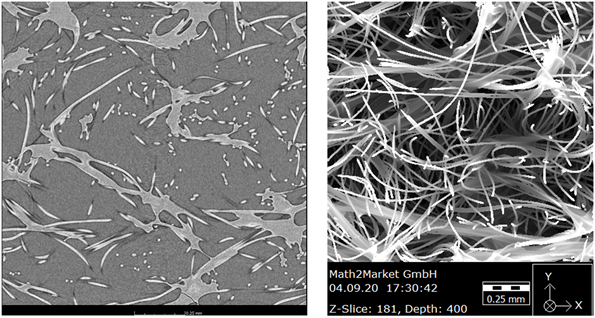Figure 1. One CT picture of Filter material and import electron microscope effect pictures

GeoDict材料分析软件可以将过滤材料电子计算机断层扫描照片，电子计算机断层扫描与计算机软件结合能够对过滤材料进行无损扫描并对图像重构，进而定量分析滤材孔隙率的变化、数目和分布 ，生成三维模型，从而进行材料特性分析如孔径分布、流电阻率(渗透性、压降)、热导率、电导率、过滤效率等。Figure 2. Three dimensional of filter material with GeoDict software

2.3. 过滤材料阻力特性的数值模拟Table 1. The simulation results of resistance properties for filter material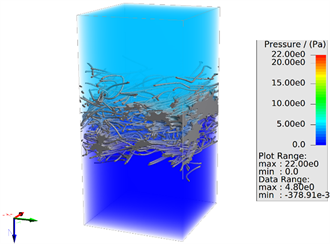Figure 3. Pressure cloud chart of filter material when the flow rate is 0.02 m∙s−1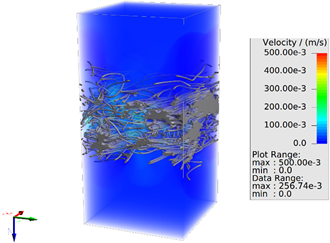Figure 4. Velocity cloud chart of filter material when the flow rate is 0.02 m∙s−1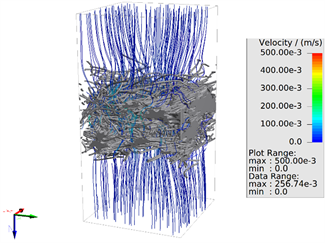Figure 5. Velocity streamline diagram of filter material along thickness direction when the flow rate is 0.02 m∙s−1

2.4. 空气过滤器的数值模拟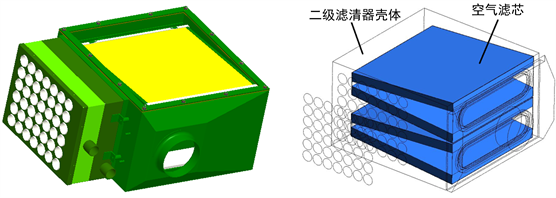Figure 6. Air filter model and simplified model

${S}_{i}=-\left(\underset{j=1}{\overset{3}{\sum }}{D}_{ij}\eta {v}_{i}+\underset{j=1}{\overset{3}{\sum }}{C}_{ij}\frac{1}{2}\rho {v}_{mag}{v}_{i}\right)$

D和C为规定的矩阵；

$\eta$ ——动力黏度，Pa·s；

vmag——速度矢量值，m·s-1

vi——i方向速度值，m·s-1

${S}_{i}=-\left(\frac{\mu }{\alpha }{v}_{i}+{C}_{2}\frac{1}{2}\rho {v}_{mag}{v}_{i}\right)$

C2——惯性阻力因子。

${S}_{i}=-{C}_{0}{|v|}^{{C}_{1}}=-{C}_{0}{|v|}^{\left({C}_{1}-1\right)}{v}_{i}$Table 2. Simulation results of resistance properties for air filter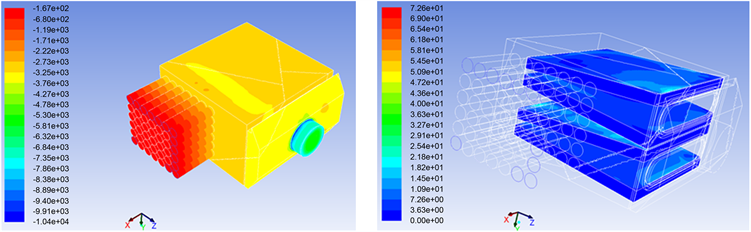Figure 7. Pressure cloud chart of air filter when the outlet flow rate is 1100 m3∙h−1

3. 仿真结果与试验结果对比

3.1. 过滤材料阻力特性试验结果Table 3. Test results of resistance properties from filter material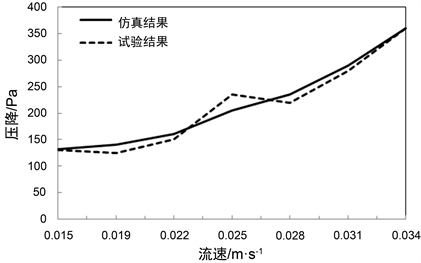Figure 8. Comparison of resistance properties for filter material between test and simulation results

3.2. 空气过滤器阻力特性试验结果Table 4. Test results of resistance properties from filter material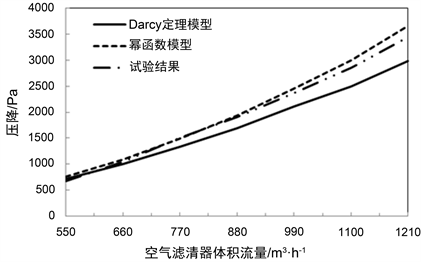Figure 9. Comparison of resistance properties for air filter between test and simulation results

4. 结论

1) 由CT照片生成三维模型，能够反映滤材的真实结构，可以进行阻力特性、效率特性等滤材性能的定量分析。

2) GeoDict材料分析软件计算得到的滤材阻力特性能够生成准确的多孔介质渗透模型，可以用于空气过滤器的数值模拟计算。

3) 在空气过滤器数值模拟中，由准确的滤材特性数据建立的幂函数多孔介质渗透模型计算结果更接近试验室结果。

4) 采用GeoDict材料分析软件结合流场分析软件的数值模拟方法能够得到准确、可信的阻力特性，可以指导空气过滤器内流场分析与性能预测。

  陈晓玉. 摩托车空气滤清器性能检测方法[J]. 摩托车技术, 2005, 4(7): 25-26.  韩青. 空气滤清器内三维紊流数值计算与分析[D]: [硕士学位论文]. 济南: 山东轻工业学院, 2008.  Fabbro, D.L. and Laborde, J.C. (2002) Air Flows and Pressure Drop Modelling for Different Pleated Industrial Filters. Filtration & Separation, 39, 34-40. https://doi.org/10.1016/S0015-1882(02)80055-6  Luckit, F.H. (1996) The Prediction of Filtration Performance of High Efficiency Gas Filter Elements. Chemical Engineering Science, 51, 1199-1208. https://doi.org/10.1016/0009-2509(95)00366-5  Subrenat, A., Bellettre, J. and Le, C.P. (2003) 3-D Numerical Simulations of Flows in a Cylindrical Pleated Filter Packed with Activated Carbon Cloth. Chemical Engineering Science, 58, 4965-4973. https://doi.org/10.1016/j.ces.2003.07.012  Nassehi, V., Hanspal, N.S., Waghode, A.N., et al. (2005) Fi-nite-Element Modelling of Combined Free/Porous Flow Regimes: Simulation of Flow through Pleated Cartridge Filters. Chemical Engineering Science, 60, 995-1006. https://doi.org/10.1016/j.ces.2004.09.073  陈乐端, 韩云龙, 钱付平. 高效空气滤清器内部气相流场的数值研究[J]. 过滤与分离, 2009, 19(1): 4-5.  罗国华, 梁云, 郑炽嵩, 等. CT扫描技术纸张结构研究中的应用[J]. 造纸科学与技术, 2006(6): 111-114.  Fluent Inc. (2013) ANSYS Fluent Users Guide. ANSYS Inc., Lebanon.  徐莉萍, 马志勇, 南晓青. 压载水过滤器流场压降模拟分析[J]. 流体机械, 2016(9): 53-56.